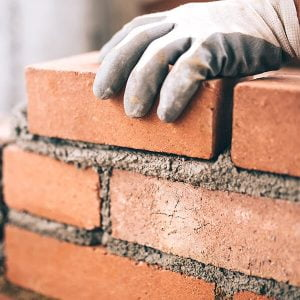Monday, October 2, 2023

# Thumb Rule for Brick Calculation | Brick Calculation in 4″, 5″, 9″, 10″, 6″ and 8″ Brick Wall

In this article, we will discuss the thumb rule for brick calculation.

### 1. Introduction

Thumb Rules are made on an experience basis that gives approximate results. It is important for civil engineers, Site engineers, or civil supervisors. It helps while taking quick decisions on construction sites.

The thumb rule for brick computation helps you compute the solution by utilizing a simple mathematical formula and making a smart decision whenever needed. It does not tell about the approximate or exact number of bricks, it provides roughly the result.

By utilizing the Thumb Rule, we can compute the approximate number of bricks needed at the construction site for your building or house, or room.There are various types of brick walls that are majorly utilized in construction are 4-inch thick brick wall is also known as single brick or half wall prepared of a single bricklayer, 9-inch thick brick wall also known as a double or full brick wall made of the double bricklayer. Bricks vary in size, so 5 inches (single brick) and 10 inches (double brick) brick walls are also popular.

You are doing the brick calculation in various units such as cubic meter (m3), cubic foot (ft3), square foot (sq ft or ft2), and square meter (sqm or m2).

### 2. Thumb Rule for Brick Calculation Per Sqm

1). Utilizing the thumb rule for brick computation in the square meters for half brick or singular layer or 4″ brick wall, multiply the length of a brick masonry wall by the height to get area measured in a square meter, then multiply that area by 50 to get the number of bricks you needed.

Then sum 10% extra for wastage and breakage during transportation storage and brick wall fixing.

No. of bricks in 4″ (single brick, half brick wall) wall per m2 = (Area in sqm × 50) + 10% extra.

2). Utilizing thumb rule for brick computation in the square meter for full brick or double layer or 9″ brick wall, multiply the length of a brick masonry wall by the height to get area calculated in a square meter, then multiply that area by 100 to get the number of bricks you needed.

Then sum 10% extra for wastage and breakage during transportation storage and brick wall fixing.

No. of bricks in 9″ (double brick, full brick wall) wall per m2 = (Area in sqm × 100) + @10% extra.

### 3. FAQ

1.  A brick wall of 5 meters in length and in 3-meter height made of 4″ thickness or single brick, half brick wall, then calculation No. of bricks you needed by using Thumb Rule for Brick Calculation.

Multiply the length and height,

= 5m ×3m

= 15m2,

here we got 15 square meter area, multiply the area with 50

= 15×50

= 750 nos,

thus you will need approximately 750 nos bricks for this brick wall.

2. A brick wall of 5 meters in length and in 3-meter height made of 9″ thickness or double brick, full brick wall, then calculation No. of bricks you needed by using Thumb Rule for Brick Calculation.

Multiply the length and height

= 5m ×3m

= 15m2,

Here we get 15 square meter area, multiply the area with 100

= 15× 100

= 1500 nos,

thus you will need approximately 1500 nos bricks for this brick wall.

 Read Also: Water Required For M20 ConcreteHe is a founder and lead author of Dream Civil International and his civil engineering research articles has been taken as source by world's top news and educational sites like USA Today, Time, The richest, Wikipedia, etc.
Latest Articles

Related Articles